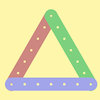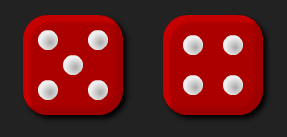#### You may also like### I'm Eight

Find a great variety of ways of asking questions which make 8.### Let's Investigate Triangles

Vincent and Tara are making triangles with the class construction set. They have a pile of strips of different lengths. How many different triangles can they make?### Noah

Noah saw 12 legs walk by into the Ark. How many creatures did he see?

# Round the Two Dice

## Round the Two DiceThere are two dice, each of them with faces labelled from 1 to 6.
When the dice are rolled they can be combined in two different ways to make a 2-digit number.

For example, if I roll a 2 and a 3 I can combine them to make 23 or 32.

Now round each of these numbers to the nearest 10:  23 rounds to 20 and 32 rounds to 30.

Repeat for other rolls of the dice.

Do both of the numbers you make ever round to the same multiple of 10?

There some interactive dice here that you can use for this problem.

#### Why do this activity?

This activity provides a meaningful task for practising rounding two-digit numbers to the nearest multiple of 10. It encourages children to record their results, notice patterns and make predictions.

#### Possible approach

A possible starting point is using a number line to remind the class what is meant by rounding.
The interactive dice can be used to model the activity on an interactive whiteboard or can be used by the children as they engage with the task. You may also find that individual dice are useful.

As the task is being modelled, the results should be recorded in a table, perhaps like the one below:

 Numbers rolled 1st two-digit number Rounds to 2nd two-digit number Rounds to 2 and 3 23 20 32 30 2 and 6 26 30 62 60

This organisation of results will support the children to notice patterns and conjecture about when numbers will round to different multiples of 10.

Some children may move onto the extension tasks (below).

#### Key questions

Which numbers can we make?
What will they round to? Will they round up or down? Why?
Do they round to the same multiple of 10? Why? When will this happen?

#### Possible extensions

Extension 1: Did the class find examples where both two-digit numbers round to the same multiple of 10? Can they then come up with a rule about when both of the two-digit numbers will round to the same multiple of 10?
Extension 2: Having completed the original task, ask the children to add a column to the right hand side of their table to note when numbers round up or down. Can they predict from the initial dice roll, whether the two-digit numbers made will round up or down?
Extension 3: What if you change the numbers on the faces of the dice?
Extension 4: What happens if you introduce a third dice and make six different two-digit numbers? Can they ever all round to the same multiple of 10?
Extension 5: Have a go at the activity Round the Three Dice and practise rounding numbers to the nearest multiple of 100.

#### Possible support

Copies of the table to write straight into would be useful.
A number line would also be helpful to support children in reasoning about which multiple of 10 is closest to a given number.